Visual Explanation of the Van-Cittert Zernike Theorem - The Double Slit Experiment with Incoherent and Coherent Light

What happens when the double slit experiment is performed with incoherent light (for example with a light bulb)? And how it differs when it is performed with coherent light (for example with a laser)?

The main idea of these simulations is to answer this question, simulating the light propagating through the double slit at different time scales: (femtoseconds, picoseconds and microseconds) to show their differences.

The topics shown in this video are discussed in Statistical Optics Books and usually treated with the Van Cittert–Zernike theorem (we'll discuss it later), but what happens it's that they are a bit mathematically obscure.

I thought that a visualization of the topic could be helpful, but I found almost zero of them both in the internet and in the literature, so this is the reason I made these simulations, to help to better understand this important topic in Optics (Coherence).

The simulations were done using the finite-difference time-domain method (FDTD) applied to Maxwell equations.

The incoherent light is simulated computing the field created by oscillating dipoles sources with random phases and wavelengths and randomly placed inside the light source dimensions (a rectangle). The dipoles represent the electronic transitions of the excited atoms of the light source.

The microseconds and picoseconds simulations are obtained when the field is averaged over that period of time.

You can find the source code of the simulations in their GitHub repository

You can change the parameters of the simulations just typing the values you want in the scripts that are indicated.

While the femtoseconds simulations only took a few minutes to be completed, the microsecond simulations 2:28 took hundreds of hours to be completed in a personal computer!

In the femtoseconds scale you can slow down the video to x0.25 in youtube settings if you find the flickering annoying. Also the video is uploaded at HD 1440p to avoid the artifacts that youtube video encoder creates with the waves at lower resolutions. You can see all fine details of the waves if you watch the video at this resolution.

Why do the interference patterns fluctuate on the picoseconds time scale?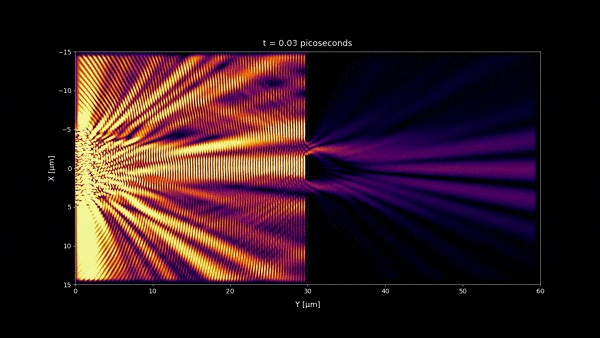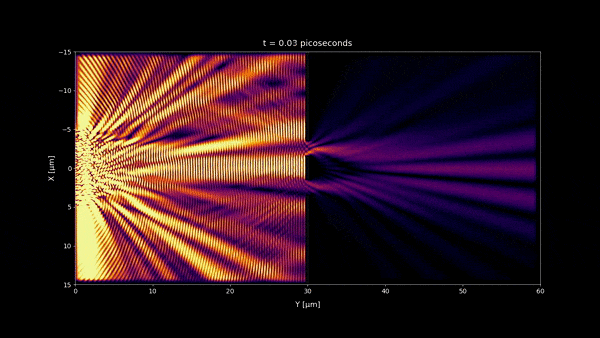Interference patterns fluctuate on picoseconds time scale because this is the order of magnitude of the coherence time of the source. This is the minimum time to make the electric field change considerably .

\begin{equation} \tau_{coh} \approx \frac{ \lambda ^2}{c \Delta \lambda} \label{eq:1} \end{equation}

where:

$$\Delta \lambda = \text{bandwidth of the light source}$$ $$\lambda = \text{center wavelength}$$ $$c = \text{speed of light}$$

In the simulation was used a wavelength of $\lambda = 650 \text{ nm}$ and a bandwidth of $\Delta \lambda = 1 \text{ nm}$. Plugging these values in the formula \eqref{eq:1} we get a coherence time of:

$$\tau_{coh} \approx 1.4 \text{ picoseconds}$$

You can see that a very narrow bandwidth $\Delta \lambda = 1 \text{ nm}$ is enough to make interferences fluctuate very fast. $\lambda = 650 \text{ nm}$ correspond to red light, and the narrow bandwidth makes almost no difference in color to the human eye.

When the intensity is averaged over a few microseconds no fluctuations can be seen. This is the reason that although the wave-like behaviour of light, we don't usually see the interferences.

More explanations: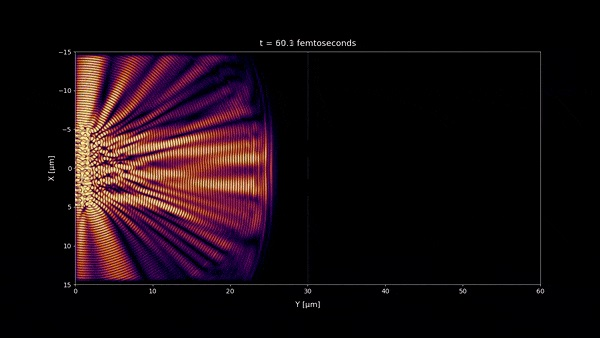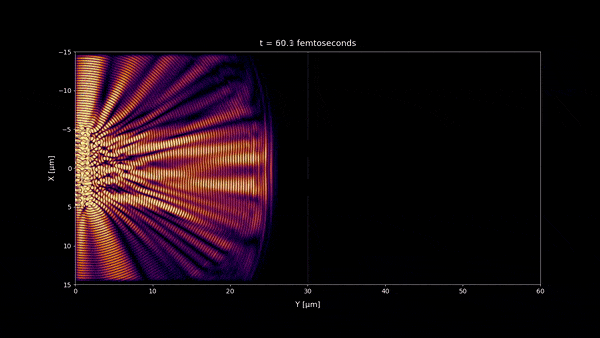• The blinking on the femtosecond time scale is because when the light is reflected on the double slit wall is due to a standing wave formed by interference from the incident and reflected waves, with an oscillation frequency equal to the frequency of the wave.

•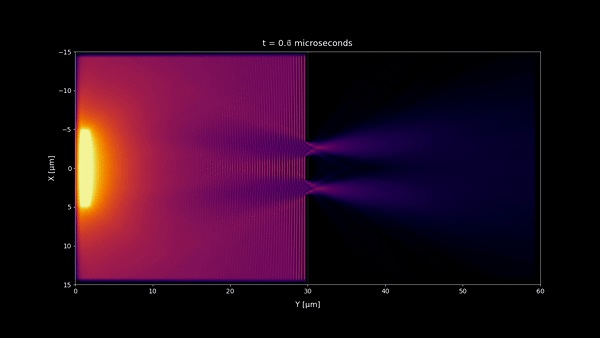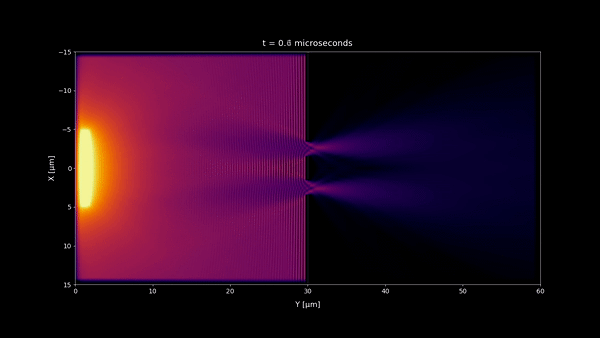• In microseconds time scale 1:20 and any longer time scale, no incoherent light interference pattern should be visible as we observe in most of our daily life. But a stationary wave is still visible in the microseconds time scale near the double slit wall. However because its size is very small , you won't notice it at macroscopic scale and instead you will see a uniform pattern. (notice that the space scale of the simulations are 60 x 30 μm)

The Van-Cittert Zernike Theorem

Finally, we comment how the irradiance patterns on the screen $I$ at the microsecond time scale can be approximated using the Van-Cittert Zernike theorem and Fraunhofer approximation:

\begin{equation} I ∝ \operatorname {sinc}^2{\left( \frac{𝜋 a x}{z \lambda}\right)} \left( 1 + \gamma \cos{\left(\frac{2𝜋D}{z\lambda} x\right)}\right) \label{eq:2} \end{equation}

where:

$$D = \text{ distance between the slits}$$ $$a = \text{ slits width}$$ $$\gamma = \text{ degree of spatial coherence}$$ $$M = \text{ width of the light source}$$ $$z = \text{ distance from the screen to the double slit}$$ $$L = \text{ distance from the light source to the double slit}$$

As Van-Cittert Zernike theorem states, $\gamma$ can be computed taking the Fourier Transform of the intensity distribution of the light source as follows:

\begin{equation} \gamma = \frac{\int\nolimits_{-\infty}^{\infty} I(x') e^{i \frac{2 \pi D}{\lambda L} x'} dx'}{\int\nolimits_{-\infty}^{\infty} I(x') dx'} \label{eq:3} \end{equation}

Using an uniform intensity distribution $I_0$ and a width of the light source of $M$:

$$\begin{gathered} I(x') = \begin{cases} I_0 & \text{ if } x' < \left|\frac{M}{2}\right| \newline 0 & \text{ if } x' > \left|\frac{M}{2}\right| \newline \end{cases} \end{gathered}$$

And computing the integrals \eqref{eq:3} we finally get the degree of spatial coherence:

\begin{equation} \gamma = \operatorname {sinc}{\left(\frac{𝜋 D M}{L \lambda}\right)} \label{eq:4} \end{equation}

Although \eqref{eq:2} doesn't produce exact results for the scale of these simulations, you can use it for qualitative predictions. When $\gamma = 1$ the fringes are perfectly visible, and when $\gamma = 0$ they cannot be seen. The further you place the light source from the double slit, the closer the coherence degree will be of $1$ .

To illustrate the equation \eqref{eq:2} we have plotted it for different degrees of coherence: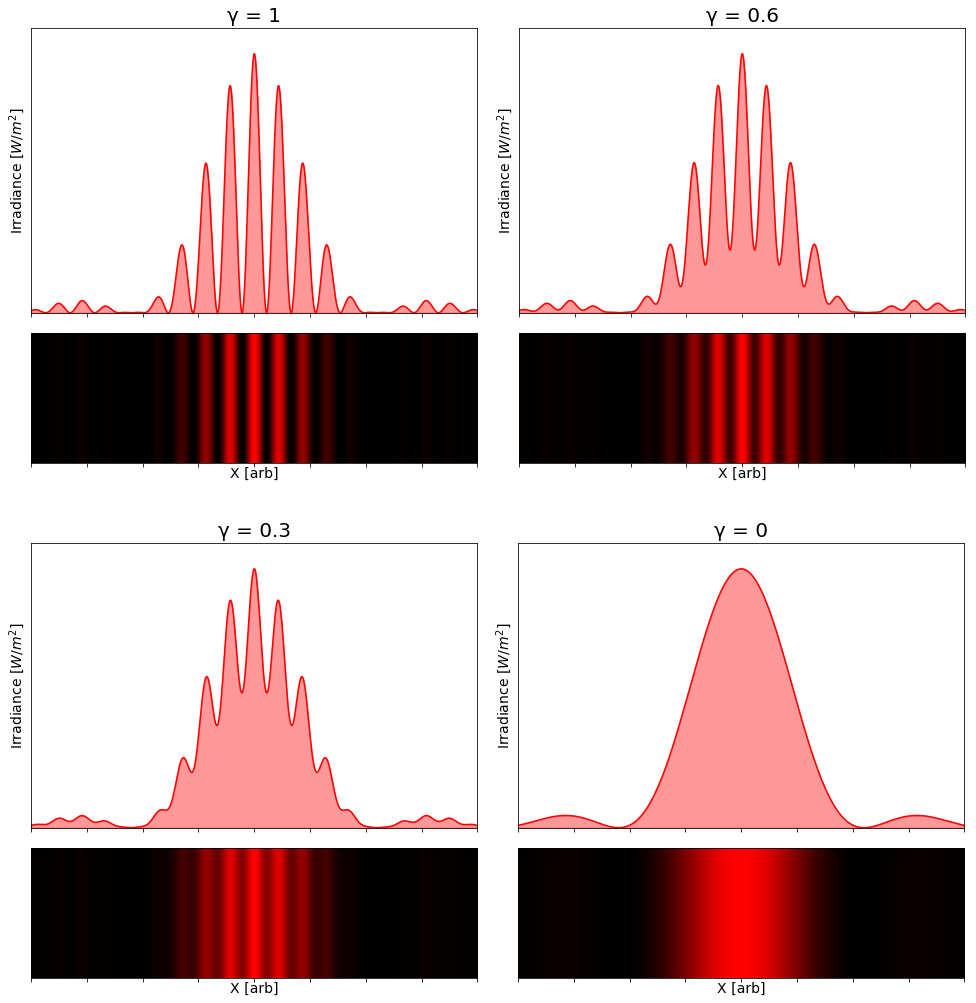This experiment is important because it's usually the easiest to set up to measure the degree of coherence of a light source. For an experimental discussion see for example . I hope these simulations have helped you to visualize how it really works.

Bibliography

 Goodman J W Statistical Optics sec. 5.1

 Brett J. Pearson, Natalie Ferris, Ruthie Strauss, Hongyi Li, and David P. Jackson, "Measurements of slit-width effects in Young’s double-slit experiment for a partially-coherent source," OSA Continuum 1, 755-763 (2018)

© 2019-2021 Rafael de la Fuente